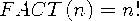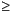Functions and CALL Routines

# FACT Function

Computes a factorial.
 Category: Mathematical

## Syntax

 FACT(n)

### Arguments

n

is a numeric constant, variable, or expression.

The mathematical representation of the FACT function is given by the following equation:with n0.

If the expression cannot be computed, a missing value is returned. For moderately large values, it is sometimes not possible to compute the FACT function.

SAS Statements Results
`x=fact(5);`
`120`

 Functions:Previous Page | Next Page | Top of Page